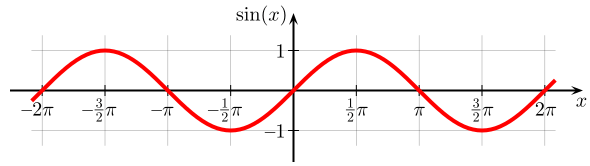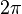# Periodic function facts for kids

Kids Encyclopedia FactsThe sine function is an example of a periodic function.

In mathematics, a periodic function is a function which has a repeated pattern (a period) which is continued infinitely.

Examples of periodic functions are the trigonometric functions sine and cosine, which both have a period of$2\pi$.Periodic function Facts for Kids. Kiddle Encyclopedia.